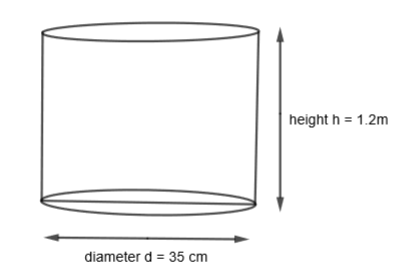QuestionAnswers

# Find the capacity (in liters) of a cylindrical storage tank of height 1.2 m and base diameter 35 cm.- Hint: First of all consider the cylinder of height 1.2m and diameter 35 cm. Now, find the radius by using $\dfrac{diameter}{2}$. Now, use the formula for finding the volume of the cylinder that is $\pi {{r}^{2}}h$ and use it to get the required answer.

Complete step-by-step solution -

In this question, we have to find the capacity of a cylindrical storage tank of height 1.2 m and base diameter 35 cm in liters. Before proceeding with the question, let us know what the capacity of a vessel means. Basically, the capacity is nothing but the volume of the vessel or the amount of the liquid or any other substance, a vessel or a container can hold. Whenever we are asked to find the capacity of a container or vessel, we need to find its volume. We generally express capacity in terms of liters, milliliters, etc.
Now, let us consider our question. Here, we are given a cylinder of 1.2m height and a diameter of 35 cm. Let us draw the cylinder to visualize the question.We know that the volume of the cylinder is $\pi {{r}^{2}}h$ where r is the radius of the cylinder and h is the height of the cylinder. We are given that the height of the cylinder = 1.2m $=1.2\times 100=120cm$
We are given that the diameter of the cylinder d = 35 cm.
We know that,
$Radius=\dfrac{Diameter}{2}$
So, we get, the radius of the cylinder,
$r=\dfrac{diameter}{2}=\dfrac{35}{2}cm$
Let us substitute the value of h = 120 cm and $r=\dfrac{35}{2}cm$ in the volume of the cylinder. So, we get,
The volume of the cylinder $=\pi {{r}^{2}}h$
$=\pi {{\left( \dfrac{35}{2} \right)}^{2}}\left( 120 \right)c{{m}^{3}}$
By substituting the value of $\pi =\dfrac{22}{7}$, we get,
The volume of the cylinder $=\left( \dfrac{22}{7} \right).\left( \dfrac{35}{2} \right)\left( \dfrac{35}{2} \right)\left( 120 \right)c{{m}^{3}}$
$=\left( 22 \right)\left( 5 \right)\left( 35 \right)\left( 30 \right)c{{m}^{3}}$
$=115500c{{m}^{3}}$
We know that,
$1\text{ }liter=1000c{{m}^{3}}$
$1c{{m}^{3}}=\dfrac{1}{1000}liters$
So, we get,
$115500c{{m}^{3}}=\dfrac{115500}{1000}=115.5liters$
So, we get the capacity of the cylinder as 115.5 liters.

Note: In this question, many students make this mistake of substituting diameter in place of the radius which is wrong. They must properly read and convert the diameter into radius by halving if and then use it. Also, in any formula, all the dimensions must be of the same unit. So, first convert all the dimensions in the same unit as we took height and radius, both in cm and then use it to get the correct answer.
Volume and CapacityTo Find the Weight of a Given Body Using Parallelogram Law of VectorsHow to Find Square Root of a NumberThe Making of a ScientistChanging the Period of a PendulumVolume of a Triangular Prism FormulaArea of a Sector of a Circle FormulaHeat Capacity FormulaMotion of a Charged Particle in Magnetic FieldElectric Field of a SphereImportant Questions for CBSE Class 6 English A Pact with The Sun Chapter 10 - A Strange Wrestling MatchImportant Questions for CBSE Class 6 English A Pact with The Sun Chapter 1 - A Tale of Two BirdsImportant Questions for CBSE Class 6 English A Pact with The Sun Chapter 8 - A Pact with the SunImportant Questions for CBSE Class 7 English An Alien Hand Chapter 9 - A Tiger In The HouseImportant Questions for CBSE Class 10 Maths Chapter 3 - Pair of Linear Equations in Two VariablesImportant Questions for CBSE Class 6 English A Pact with The SunImportant Questions for CBSE Class 7 English Honeycomb Chapter 10 - The Story of CricketImportant Questions for CBSE Class 6 English Honeysuckle Chapter 8 - A Game of ChanceImportant Questions for CBSE Class 11 Business Studies Chapter 7 - Formation of a CompanyImportant Questions for CBSE Class 6 English A Pact with The Sun Chapter 2 - The Friendly MongooseCBSE Class 10 Hindi A Question Paper 2020Hindi A Class 10 CBSE Question Paper 2009Hindi A Class 10 CBSE Question Paper 2015Hindi A Class 10 CBSE Question Paper 2016Hindi A Class 10 CBSE Question Paper 2012Hindi A Class 10 CBSE Question Paper 2010Hindi A Class 10 CBSE Question Paper 2008Hindi A Class 10 CBSE Question Paper 2014Hindi A Class 10 CBSE Question Paper 2007Hindi A Class 10 CBSE Question Paper 2013RD Sharma Solutions for Class 9 Maths Chapter 19 - Surface Area and Volume of A Right Circular CylinderNCERT Solutions for Class 10 English Footprints Without Feet Chapter 6 - The Making of a ScientistNCERT Solutions for Class 10 Social Science India and the Contemporary World - II Chapter 4 - The Making of a Global WorldRS Aggarwal Class 8 Mathematics Solutions for Chapter-18 Area of a Trapezium and a PolygonNCERT Solutions for Class 10 English Footprints Without Feet Chapter 4 - A Question of TrustRD Sharma Solutions for Class 9 Maths Chapter 21 - Surface Area and Volume of a SphereNCERT Solutions for Class 12 Accountancy Chapter 4 - Reconstitution of a Partnership Firm:Retirement/Death of a PartnerNCERT Solutions for Class 12 Accountancy Chapter 3 - Reconstitution of a Partnership Firm: Admission of a PartnerNCERT Solutions for Class 6 English A pact with the Sun Chapter-10RS Aggarwal Class 11 Solutions Chapter-16 Conditional Identities Involving the Angles of a Triangle